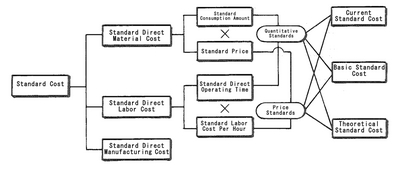#### Asprova MRP

Asprova MRP is calculated on the memory requirement, allocate lead time at a high speed. Can achieve material arrangement Just in Time, which is impossible in other MRP system.

MRP glossary TOP > S > Standard Cost Accounting

## Standard Cost Accounting

Standard Cost Accounting

It refers to cost accounting which is performed in a scientific and statistical manner using the standard consumption amount and standard price. This calculation is done to thoroughly control the cost and improve the efficiency of management, while actual cost method using actual consumed amount and actual price is done for financial accounting. To perform standard cost accounting, the standard cost should be first set. The standard cost can be divided into three by the period to be covered and the degree of goal: Current Standard Cost, Basic Standard Cost, and Theoretical Standard Cost. As for direct material cost, direct labor cost, and indirect manufacturing cost, the following standard is set for each:

Standard Direct Material Cost = Standard Consumption Amount x Standard Price
Standard Direct Labor Cost = Standard Direct Operating Time x Standard Labor Cost per Hour
Standard Direct Manufacturing Cost: either Period Budget or Variable Budget

The relationship among these standard costs is shown in the figure, and the cost control is mainly for quantitative standards by performing the standard cost accounting.Related term: Cost Accounting

Reference：JIT Business Research Mr. Hirano Hiroyuki